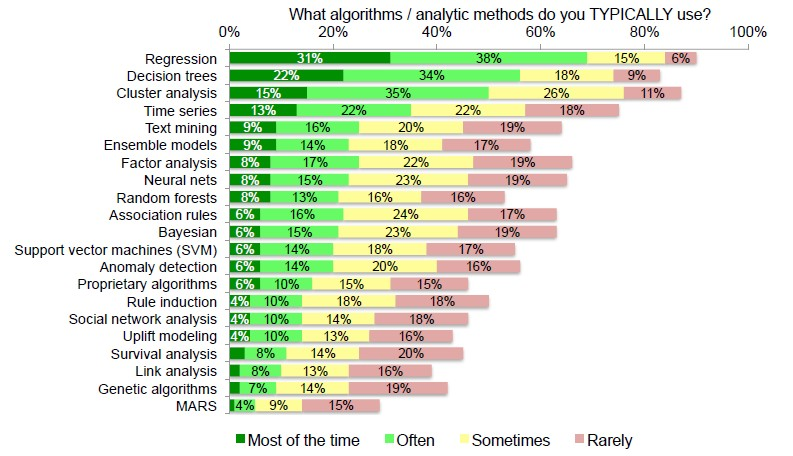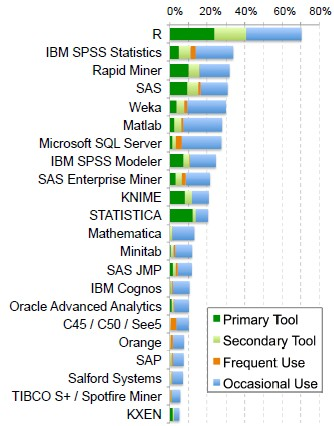# Machine Learning - (Probabilistic?) Neural Network (PNN)

Neural network was borned to resolve the problem of handwritten digits recognition taken from envelopes.

A neural network with a hidden layer has universality: given enough hidden units, it can approximate any function (???)

## Documentation / Reference

Recommended Pages(Machine learning|Inverse problems) - Regularization

Regularization refers to a process of introducing additional information in order to: solve an ill-posed problem or to prevent overfitting. This information is usually of the form of a penalty...Data Mining - (Discriminative|conditional) models

Discriminative models, also called conditional models, are a class of models used in machine learning for modeling the dependence of an unobserved variable y on an observed variable x. Discriminative...Data Mining - Algorithms

An is a mathematical procedure for solving a specific kind of problem. For some data mining functions, you can choose among several algorithms. Algorithm Function Type Description Decision...Keras

is a high-level neural networks API developed with a focus on enabling fast experimentation. Recognizing handwritten digits from the MNIST_databaseMNIST dataset. . See ...Machine Learning

Systems that automatically learn programs from data [Domingos 2012] Teaching a computer the world [Mark Dredze] “”“”Gwen Shapira NIVOZ...Machine Learning - Deep Learning (Network)

Deep Learning (Networks) is an algorithms which is basically neural networks with many layers. Deep learning is also known as: deep machine learning, deep structured learning, hierarchical learning,...Time Serie - Forecasting (Prediction)

Forecasting is the process of making predictions of the future based on past and present data (time serie) and analysis of trends. Prediction is a similar, but more general term. Usage can differ...